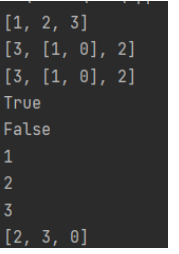# Python基础07 函数

+关注继续查看

### 函数的定义

def square_sum(a,b):
c = a**2 + b**2
return c

c = a**2 + b**2        # 这一句是函数内部进行的运算

return c               # 返回c的值，也就是输出的功能。Python的函数允许不返回值，也就是不用return。

return可以返回多个值，以逗号分隔。相当于返回一个tuple(定值表)。

return a,b,c          # 相当于 return (a,b,c)

### 函数调用和参数传递

print square_sum(3,4)

Python通过位置，知道3对应的是函数定义中的第一个参数a， 4对应第二个参数b，然后把参数传递给函数square_sum。

（Python有丰富的参数传递方式，还有关键字传递、表传递、字典传递等，基础教程将只涉及位置传递）

a = 1

def change_integer(a):
a = a + 1
return a

print change_integer(a)
print a

#===(Python中 "#" 后面跟的内容是注释，不执行 )

b = [1,2,3]

def change_list(b):
b = b + 1
return b

print change_list(b)
print b

### 总结

def function_name(a,b,c):
statement
return something  # return不是必须的

return     NonePython基础篇：函数的深入简出
Python基础篇：函数的深入简出
4 027 019 0Python基础之:函数
Python基础之:函数
23 0python基础之函数

760 0Python之路【3】：Python基础（二） 函数的理解

1459 0Python基础（7）--函数
1218 0Python基础 3----Python函数及类
1 函数的定义和调用     定义函数     def 函数名(参数列表):            函数体     函数调用：函数名(参数列表)           2 Python的变长参数，变长参数会被自动的转变为以...
1021 0Python基础07 函数

822 0python——基础练习（三）
python——基础练习（三）
20 0+关注

10424

2